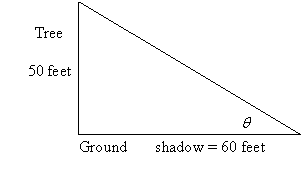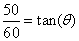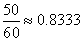Friday, October 9, 2009

Angles of Elevation and Depression

This is the last topic I taught before the final exam. This is a very simple topic that can be taught in one sitting. It involved trigonometry which the students are exposed to in lower forms.

The angle of elevation of an object as seen by an observer is the angle between the horizontal and the line from the object to the observer's eye (the line of sight).If the object is below the level of the observer, then the angle between the horizontal and the observer's line of sight is called the angle of depressionWord Problems: Angles of Elevation and Depression
In order to solve problems involving angles of elevation and depression, it is necessary to

* use basic right triangle trigonometry
* solve equations which involve one fractional term is also important to know.
* find an angle given a right triangle ratio of sides.
* the fact that corresponding angles formed by parallel lines have the same measure.

A typical problem of angles of elevation and depression involves organizing information regarding distances and angles within a right triangle. In some cases, you will be asked to determine the measurement of an angle; in others, the problem might be to find an unknown distance.

Suppose a tree 50 feet in height casts a shadow of length 60 feet. What is the angle of elevation from the end of the shadow to the top of the tree with respect to the ground?

First we should make a diagram to organize our information. Look for these diagrams to involve a right triangle. In this case, the tree makes a angle 90º with the ground. A diagram of this right triangle is shown below.In the diagram, known distances are labeled. These are the 50 and 60 foot legs of the right triangle corresponding to the height of the tree and the length of the shadow.

The variable q is chosen to represent the unknown measurement, the object of the question.

To relate the known distances and the variable, an equation is written. In this case the equation involves the lengths of the sides which are opposite and adjacent to the angle q. Using the ratio of opposite to adjacent sides, we haveWe use inverse tangent oforwhich is the angle of elevation.

Watch Video on Word Problems on Angle of Elevation & Depression